Scroll

# Listing Several Aggregated Calculations in a Variable

Follow
 Summary This article is one in a series of articles that will go through examples of using aggregate script syntax new to Askia 5.3.5.0. Specifically, this article demonstrates how to list a number of statistical tests in one calculated variable. It also give examples of how to use the PreviousQuestion, NextQuestion, Max(), Min(), MaxValue, MinValue, ToString() and Format() keywords Applies to Askia Analyse. Written for Researchers, Data Processors, Analysts. Keywords Aggregated script, Calculated variables, Numeric by script, Find all values of a script, Data object, Value, Counts, Create bands, PreviousQuestion, NextQuestion, Max(), Min(), MaxValue, MinValue, ToString(), Format(), MinDate, MaxDate.

The .qes file and portfolio containing the example discussed is attached: Aggregated Scripts Examples 4.rar

You can list a number of calculations in the code-frame of a calculated variable like this: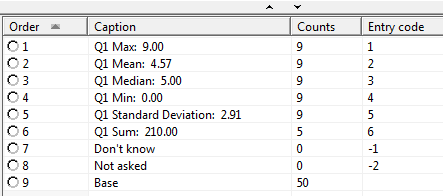Firstly, you have to make sure you have a single coded variable that always has a number of codes greater than or equal to the number of calculations you want to list:

Here it is 6. I have a numeric question ‘IntPos’ which contain unique number for each of the 50 respondents (1 to 50). I know that I can split this into six codes if I create bands of width 9. The counts going into each code are not important for the purposes of what I am trying to achieve here.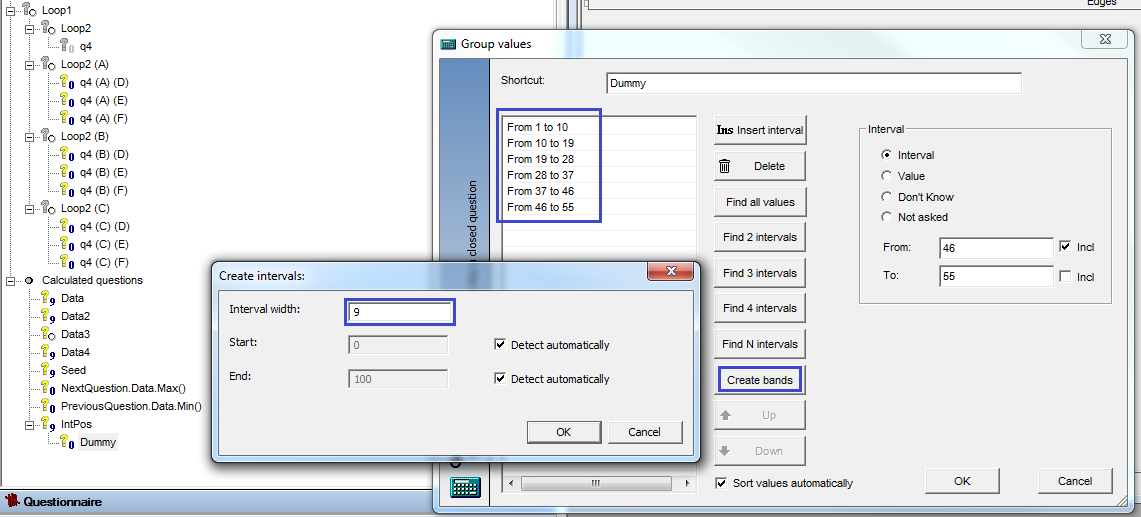Now I can set values into each of the six codes of this variable in a new created variable by using the following syntax.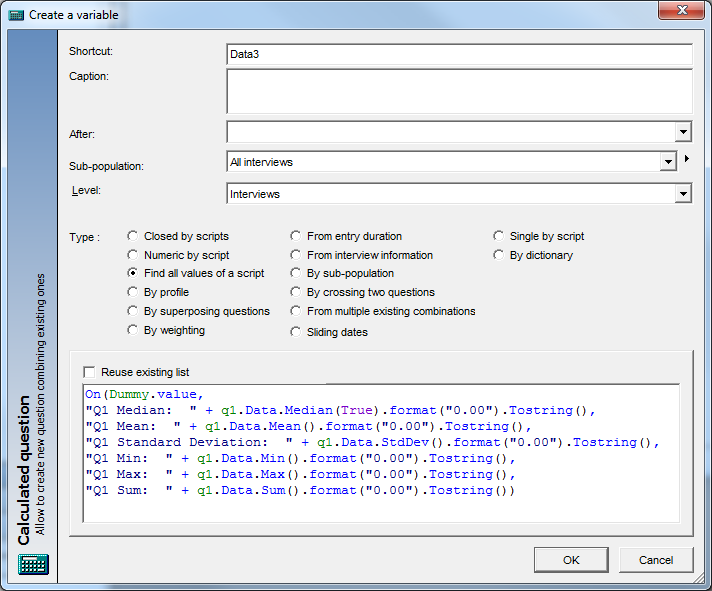.format("0.00")

This syntax is to make sure everything comes out to 2 decimal places.

.Tostring()

This syntax is to make sure everything being returned is of the same type: text.

The benefit of a variable like this is you can give yourself a quick view of some key numbers without having to tabulate variables and pick calculations. It's mainly a demonstration of what you can do with the syntax.

Let’s now demonstrate the NextQuestion and PreviousQuestion keywords:

You can probably guess what they do, they access the next or previous questions in the question tree (including created variables). Below I’m accessing the next question after Data4 in the question tree (Seed) and returning its maximum value.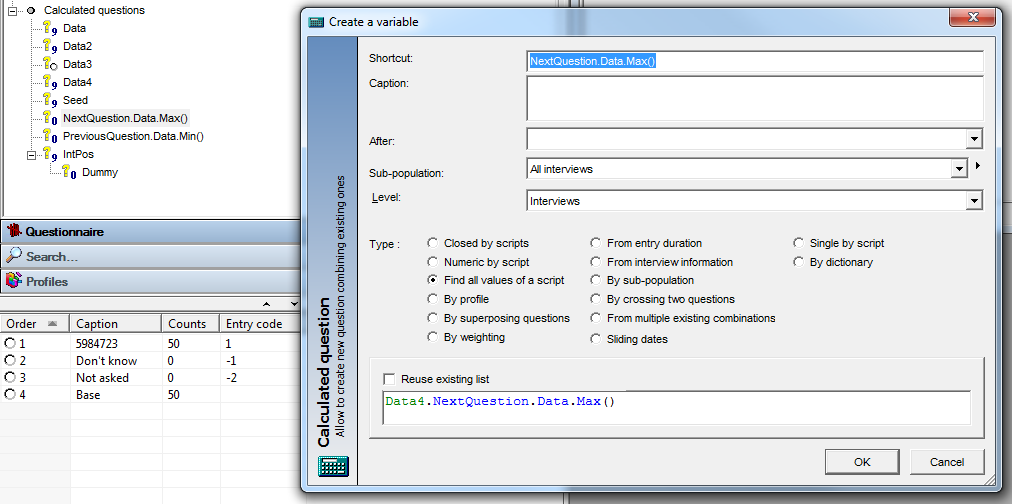In PreviousQuestion.Data.Min() I’m using the following syntax to return the minimum value stored in Seed (68585):

^NextQuestion.Data.Max()^.PreviousQuestion.Data.Min()

You may have seen the keywords MinValue & MaxValue in the syntax documentation and wondered what these do:

Well, these simply access the lower and upper limits of numeric questions.

"Q1 Lower Bound:  "+q2.PreviousQuestion.MinValue.ToString()+" / Q1 Upper Bound:  "+q2.PreviousQuestion.MaxValue.ToString()

Using the above syntax in a ‘Find all values of a script’ created variable, you will get the below.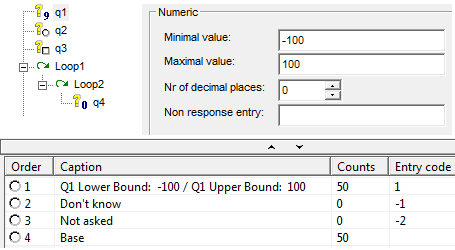The equivalent of these keywords for date variables are: MinDate & MaxDate.

Finally, let's take a look at the response and caption keywords:

If necessary we can access the variable names, their captions and properties for the response like caption and scaled value. Here are examples of the syntax you can use for this: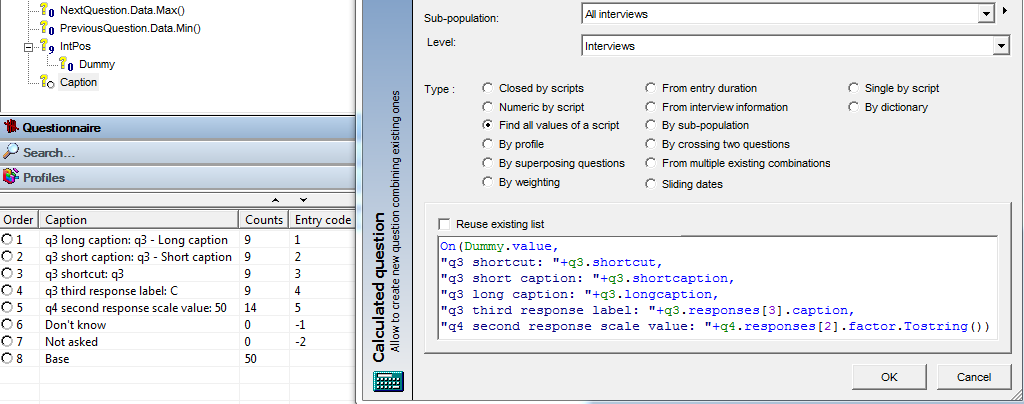This is found in the calculated variable 'Caption'.